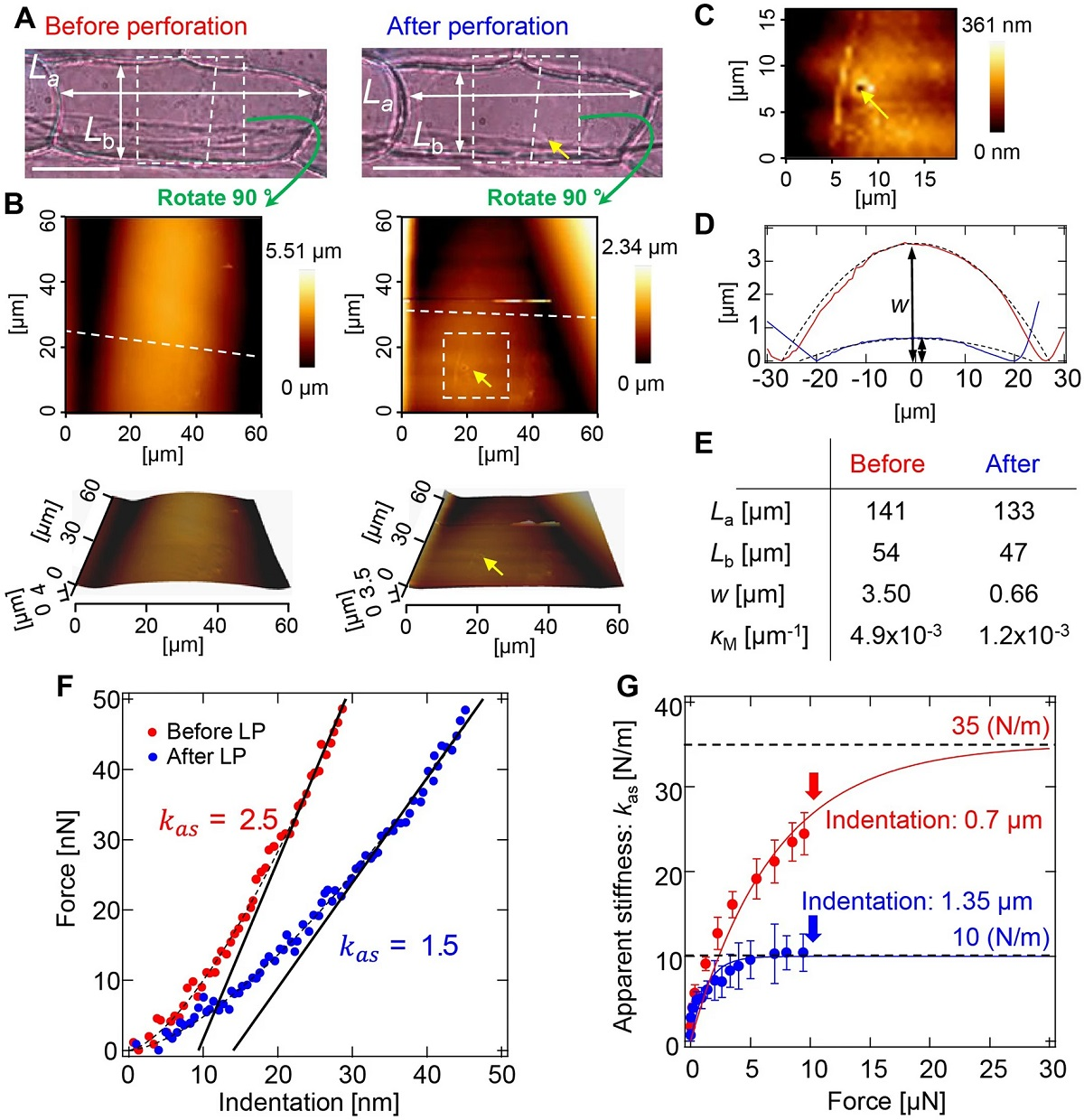# Elastic shell theory for plant cell wall stiffness reveals contributions of cell wall elasticity and turgor pressure in AFM measurement

The stiffness of a plant cell in response to an applied force is determined not only by the elasticity of the cell wall but also by turgor pressure and cell geometry, which affect the tension of the cell wall. Although stiffness has been investigated using atomic force microscopy (AFM) and Young’s modulus of the cell wall has occasionally been estimated using the contact-stress theory (Hertz theory), the existence of tension has made the study of stiffness more complex. *

An alternative model is a contact model based on elastic shell theory, in which the cell wall is assumed to be a thin, curved surface pushed by turgor pressure. This theory enables one to infer turgor pressure from the apparent stiffness in some cases. In the unified formula from the elastic shell theory, AFM indentation is described as the contributions of cell wall elasticity and turgor pressure, while the estimation of elasticity and pressure remains ambiguous. *

In the article “Elastic shell theory for plant cell wall stiffness reveals contributions of cell wall elasticity and turgor pressure in AFM measurement” Satoru Tsugawa, Yuki Yamasaki, Shota Horiguchi, Tianhao Zhang, Takara Muto, Yosuke Nakaso, Kenshiro Ito, Ryu Takebayashi, Kazunori Okano, Eri Akita, Ryohei Yasukuni, Taku Demura, Tetsuro Mimura, Ken’ichi Kawaguchi and Yoichiroh Hosokawa describe how they used finite element method simulations to verify the formula of the elastic shell theory for onion (Allium cepa) cells and further optimized the formula to analyze the apparent stiffness observed from the AFM measurement based on the elastic shell theory.*

The authors applied the formula and simulations to successfully quantify the turgor pressure and elasticity of a cell in the plane direction using the cell curvature and apparent stiffness measured by atomic force microscopy. They conclude that tension resulting from turgor pressure regulates cell stiffness, which can be modified by a slight adjustment of turgor pressure in the order of 0.1 MPa. This theoretical analysis reveals a path for understanding forces inherent in plant cells. *

NANOSENSORS™ sphere AFM probes SD-Sphere-NCH-S from the NANOSENSORS™ Special Developments List were used for the force-indentation curve measurements described in the article. NANOSENSORS™ tipless AFM cantilevers of the TL-NCH type were used to evaluate the tip radius dependence. *Figure 3 from “Elastic shell theory for plant cell wall stiffness reveals contributions of cell wall elasticity and turgor pressure in AFM measurement” by Satoru Tsugawa et al.:
AFM measurement of an onion epidermal cell with laser perforation. (A) Photographs of the cell measured before (left) and after (right) perforation. Yellow arrow indicates the perforation point. Cell lengths along long- and short- axes are denoted by La and Lb, respectively. Bars, 50 µm. (B) Topographic images before (left) and after (right) perforation. Measurement area corresponds to the dashed box area in (A). Lower images are three-dimensional images of upper images. (C) Enlarged image of the perforation point. (D) Cross-sectional graph of the cell wall surface before (red line) and after (blue line) perforation, corresponding to the height of dashed lines in upper-left and -right images in (B), respectively. Bulge height of the cell surface is denoted by w. Dashed lines are curves for curvature calculated from Lb and w. (E) Quantities determined from AFM measurement. Mean curvature of the cell wall surface κM is calculated from La, Lb, and w. (F) Force–indentation curves of the cell wall before (red dots) and after (blue dots) perforation. Dashed lines are fitting curves by the Hertz model and solid lines are fitting lines by the shell model. (G) Apparent stiffness kas as a function of force F applied to the cell wall before (red dots) and after (blue dots) perforation. kas is estimated by linear least squares fitting of the force-indentation curve in the vicinity of the F, as shown in (F). Bars on dots represent root mean squared error. Solid lines are exponential plateau curves: kas = 35 × {1 − exp(− F/7)} (red line); kas = 10 × {1 − exp(− F/1.28)} (blue line).
Abstract
The stiffness of a plant cell in response to an applied force is determined not only by the elasticity of the cell wall but also by turgor pressure and cell geometry, which affect the tension of the cell wall. Although stiffness has been investigated using atomic force microscopy (AFM) and Young’s modulus of the cell wall has occasionally been estimated using the contact-stress theory (Hertz theory), the existence of tension has made the study of stiffness more complex. Elastic shell theory has been proposed as an alternative method; however, the estimation of elasticity remains ambiguous. Here, we used finite element method simulations to verify the formula of the elastic shell theory for onion (Allium cepa) cells. We applied the formula and simulations to successfully quantify the turgor pressure and elasticity of a cell in the plane direction using the cell curvature and apparent stiffness measured by AFM. We conclude that tension resulting from turgor pressure regulates cell stiffness, which can be modified by a slight adjustment of turgor pressure in the order of 0.1 MPa. This theoretical analysis reveals a path for understanding forces inherent in plant cells.

*Satoru Tsugawa, Yuki Yamasaki, Shota Horiguchi, Tianhao Zhang, Takara Muto, Yosuke Nakaso, Kenshiro Ito, Ryu Takebayashi, Kazunori Okano, Eri Akita, Ryohei Yasukuni, Taku Demura, Tetsuro Mimura, Ken’ichi Kawaguchi and Yoichiroh Hosokawa
Elastic shell theory for plant cell wall stiffness reveals contributions of cell wall elasticity and turgor pressure in AFM measurement
Nature Scientific Reports volume 12, Article number: 13044 (2022)
DOI: https://doi.org/10.1038/s41598-022-16880-2